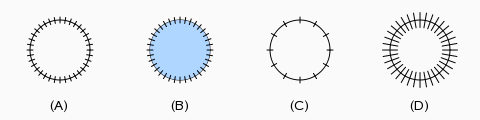# OEDrawStitchCircle¶

bool OEDrawStitchCircle(OEDepict::OEImageBase &image,
const OEDepict::OE2DPoint &center, double radius,
const OEDepict::OEPen &pen, double patternAngle=10.0,
double patternWidthRatio=0.20)


Draws a circle with the OECircleStyle::Stitch style.OEDrawStitchCircle

image
The image on which the circle is drawn.
center
The center of the circle.
radius
The radius of the circle.
pen

The graphical properties of the circle.

See examples (A) and (B) in Figure: OEDrawStitchCircle.

patternAngle

Specifies the angle (in degrees) between the patterns of the circle. The angle has to be in a range of $$[1.0^{\circ} - 90.0^{\circ}]$$

See example (C) in Figure: OEDrawStitchCircle.

patternWidthRatio

Specifies the width of the pattern of the circle relative to its radius. The ratio has to be in a range of $$[0.01 - 1.00]$$.

See example (D) in Figure: OEDrawStitchCircle.